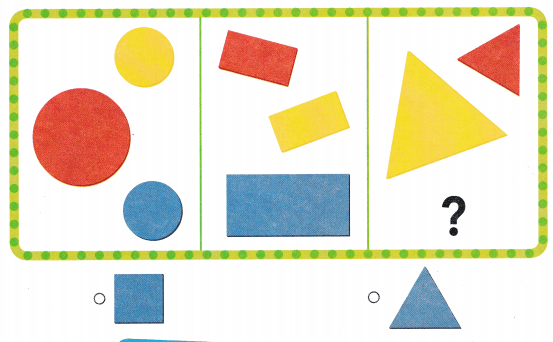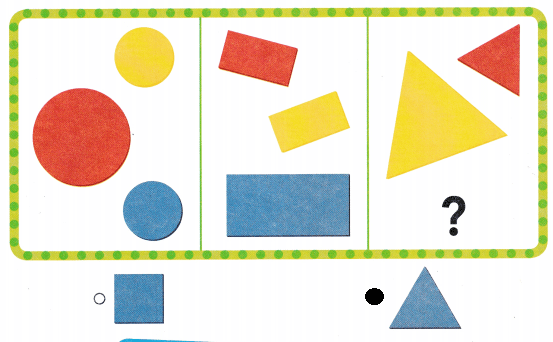# Texas Go Math Kindergarten Lesson 20.2 Answer Key Sort into Three Groups

Refer to our Texas Go Math Kindergarten Answer Key Pdf to score good marks in the exams. Test yourself by practicing the problems from Texas Go Math Kindergarten Lesson 20.2 Answer Key Sort into Three Groups.

## Texas Go Math Kindergarten Lesson 20.2 Answer Key Sort into Three Groups

Essential Question
How can you sort objects into three categories?

Explore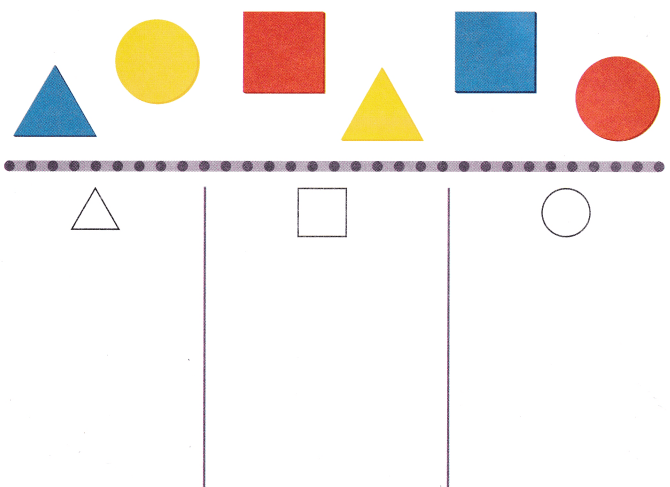Explanation:
In first row there are triangles
in the second row there are squares and in third row there are circles.

Directions
Use shapes like those shown at the top of the page. Sort them by shape. Draw and color the shapes to show how you sorted.

Share and Show

Question 1.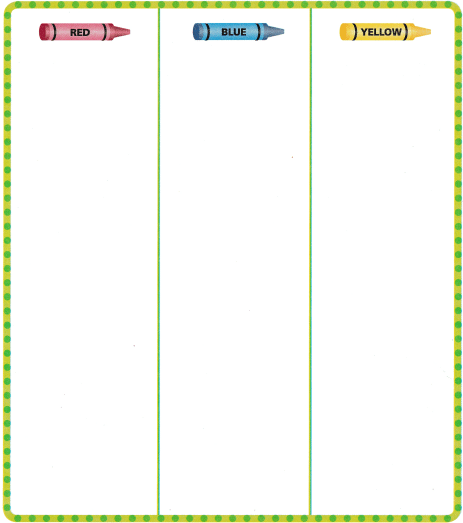Explanation:
Square, triangle and circles are sorted according to the colors
each color has 3 shapes

Directions
1. Take a handful of small shapes. Sort them by color. Draw and color the shapes to show how you sorted.

Question 2.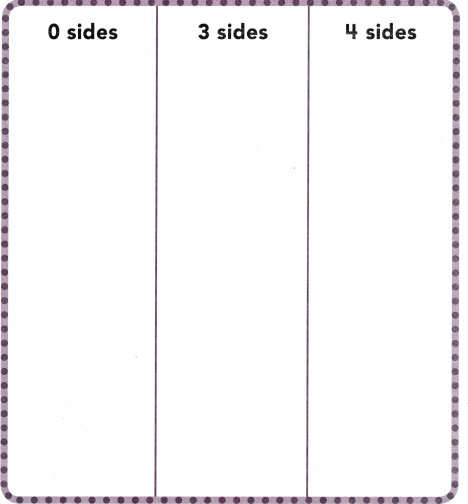Explanation:
Circle has no sides
Triangle has 3 sides
and a square has 4 sides all the sides are equal.

Directions
2. Take a handful of small shapes. Sort them by their number of sides. Draw and color the shapes to show how you sorted.

Home Activity

• Ask your child to explain how he or she sorted shapes on this page. Draw a square, a circle, or a triangle. Ask your child which category this shape would go in and why.

Problem Solving

Question 3.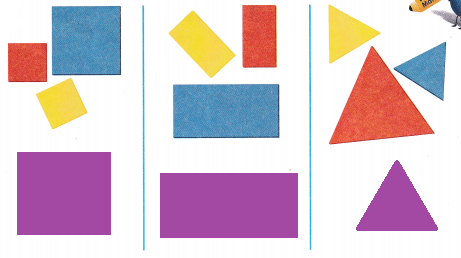Explanation:
One more square is drawn in squares column
rectangle in rectangle column and
triangle in  the triangle column respectively

Question 4.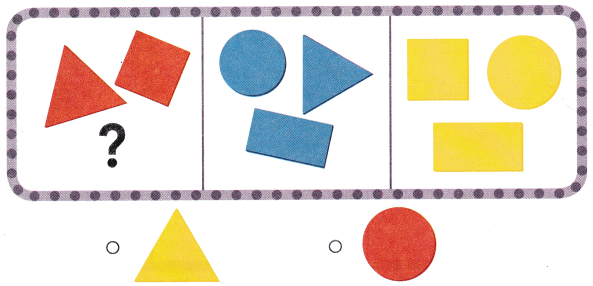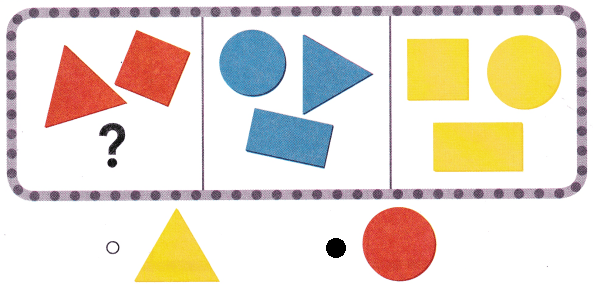Explanation:
In the red color box circle is missing
so, circle is marked.

Directions
3. How are the shapes sorted? Draw one more shape in each category. 4. Choose the correct answer. Look at how the shapes are sorted. Which shape belongs in the first category?

### Texas Go Math Kindergarten Lesson 20.2 Homework and Practice

Question 1.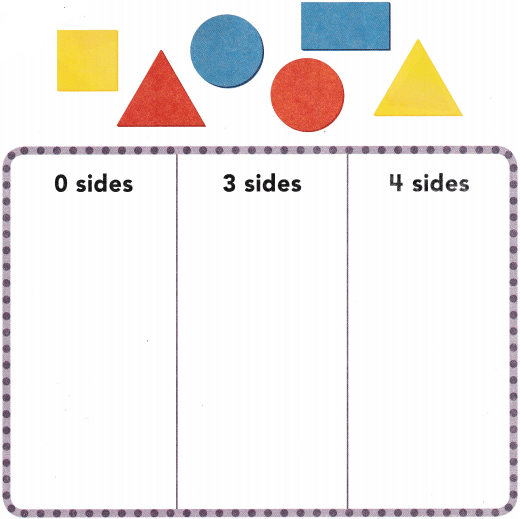Explanation:
Circle has no sides
a triangle has 3 sides
and a square has 4 sides and all sides are equal.

Directions
1. Sort the shapes at the top of the page by their number of sides. Draw and color the shapes to show how you sorted.

Texas Test Prep

Question 2.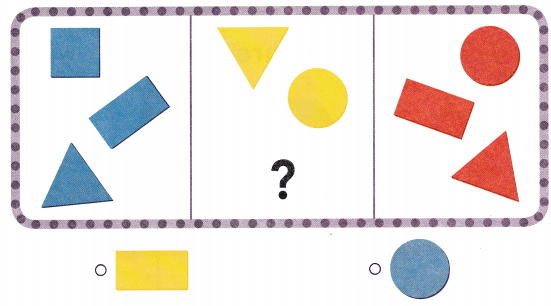Explanation:
In yellow color box a rectangle is missing
so, yellow rectangle is placed.

Question 3.